# Q value of a Nuclear reaction

The Q value of a Nuclear reaction is the energy either absorbed or released during a nuclear reaction. Let us consider a nuclear reaction,

a + X -> Y + b+ Q

in which a projectile particle 'a' having rest mass 'ma' moving with velocity va bombards with the target nucleus X having rest mass Mx at rest (Vx= 0). Then a product nucleus Y having rest mass My moves with velocity (Vy =0) along with the emission of product particle 'b'having rest mass mb moving with velocity vb.

The total energy (rest mass energy + Kinetic energy) is conserved during a nuclear reaction. So,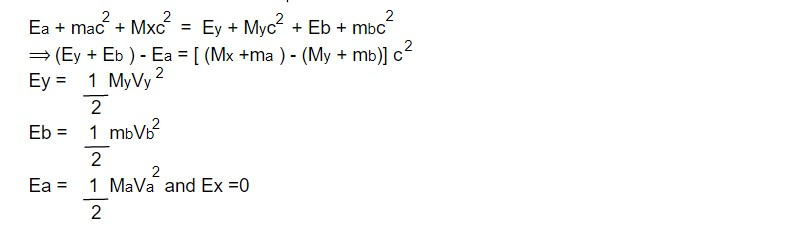The Q value of nuclear reaction can also be defined as the difference of Kinetic energy of products and that of reactants. It is the energy equivalent to mass lost during a nuclear reaction.

Exothermic nuclear reaction:

The nuclear reaction in which Q value is positive i.e energy is released then the reaction is said to be exothermic or Endoergic reaction. For Q to be positive,

`Ey + Eb - Ea = + ve`

`=> Ey + Eb > Ea`

The sum of kinetic energy of products must be greater than that of reactants or the sum of rest masses of reactants must be greater than that of products.

Endothermic or Endoergic nuclear reaction:

The nuclear reaction in which Q value is negative or energy has to be supplied for the reaction to take place is known as endoergic reaction.

For Q to be negative

`Ey + Eb - Ea = - ve`

`=> Ey + Eb < Ea`

The sum of Kinetic energy of reactant must be greater than that of products or the sum of masses of products must be greater than that of reactants.

Expression of Q value:

For expression of Q value, we have,

Q = Ey + Eb - Ea...........i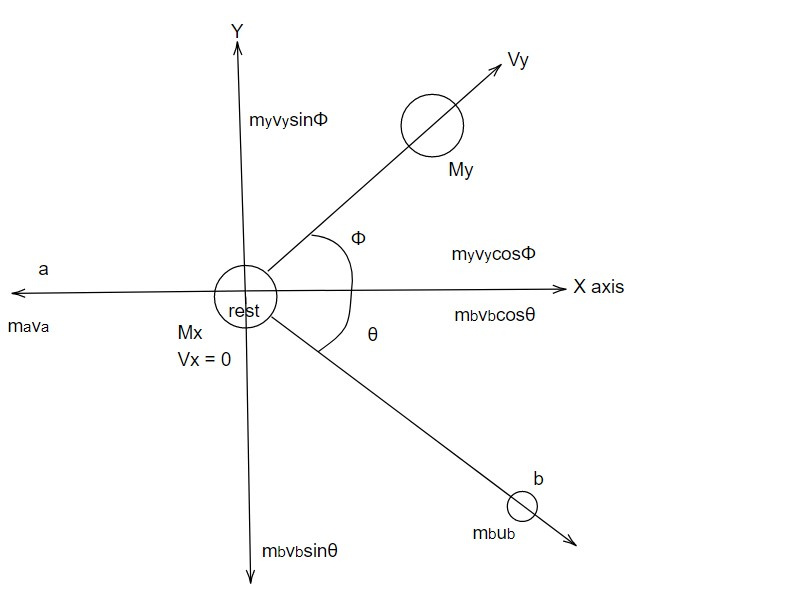Let the product nucleus Y and product particle 'b' scatter by making angle
Φ and θ respectively with incident direction of projectile particle along x axis.

Since kinetic energy of product nucleus Ey is very less and unmeasurable and cannot be measured experimentally, it is removed by using conservation laws.

From conservation of linear momentum,

Along x axisAlong y axis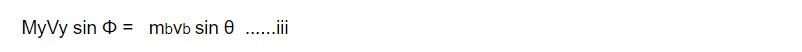Squaring and adding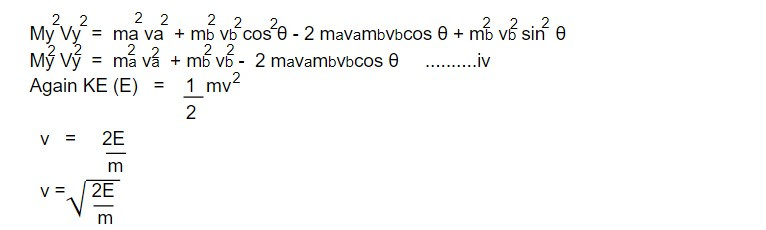Replacing V in iv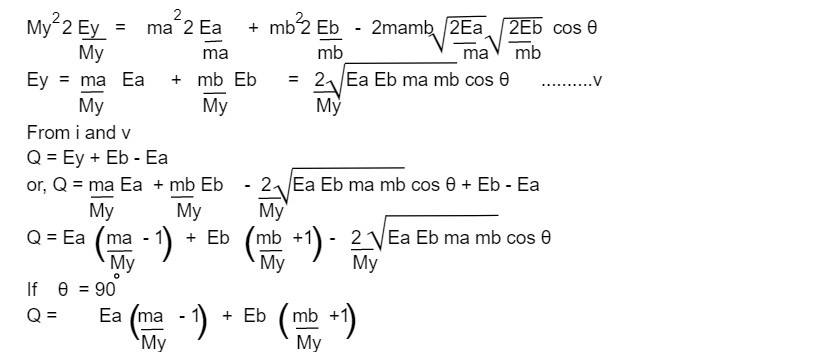Threshold Energy (Eth):

In any endoergic nuclear reaction, energy has to be supplied in the form of Kinetic energy of projectile particle for the reaction to take place. But all of the energy given to the reaction is not available. Since some part of it is used up imparting momentum to the compound nucleus which is shared by its constituent nucleons.

So, the extra energy in addition to Q value of a nuclear reaction that has to be supplied for the reaction to occur is known as threshold energy or it is the minimum Kinetic energy of projectile particle required to make nuclear reaction possible.

Let us consider a nuclear reaction in which a projectile particle 'a' having rest mass 'm ' and moving with velocity Va collides with the target nucleus 'X' having rest mass Mx at rest (Vx=0). Then a compound nucleus 'C' is formed having rest mass 'Mc' and moving with velocity Vc

`a + X -> C * -> Y + b`

Then from conservation of linear momentum

mava = McVc

Vc = maVa/ Mc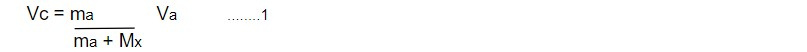Now, Q value of the reaction is defined as,

Q = Ec - Ea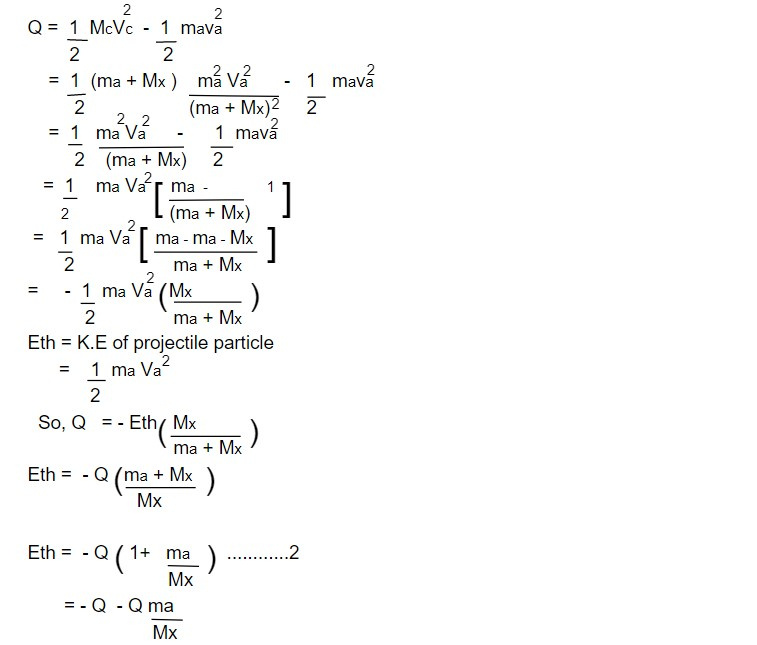So, Eth = - (Q + ∆Q)

If the projectile particle is γ ray

Rest mass of γ ray (proton) Mγ = 0

`Eth = - Q`

Types of Nuclear reaction

The process in which new particles are formed along with nucleus when the target nucleus is bombarded with the projectile particles.

The first nuclear reaction was carried by Rutherford in which proton was produced when the nucleus of Nitrogen was struck by alpha particle. According to the reaction

The reaction can also be represented as

1. Elastic scattering: The Nuclear interaction in which the projectile particle only deviates its path without any loss of energy is known as elastic scattering.

The scattering of alpha particles by gold nucleus in Rutherford experiment is elastic scattering type of the reaction.In general , the reaction can be written as

a + X -> X + a

2. Inelastic scattering: The reaction in which Kinetic energy is not conserved since some part of kinetic energy of incident particle is absorbed by target nucleus which goes to high energy quantum states and immediately returns to its ground state by emitting excess energy in the form of γ radiation. For eg: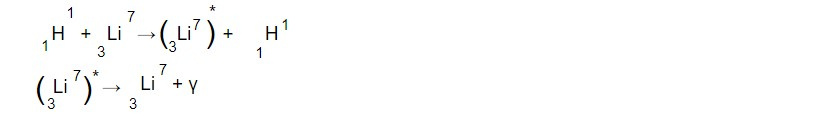In general, a + X -> X* + a

3. Disintegration reaction: The nuclear reaction in which the new product nucleus is formed along with different product particle when the target nucleus is bombarded by a projectile particle. In general,

a + X -> Y + b. For example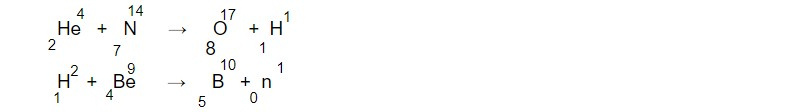4. Photo disintegration: The nuclear reaction in which the nucleus is disintegrated by absorbing energy from γ ray photon.

The energy of γ ray must be greater than B. E per nucleon of the nucleus. For example: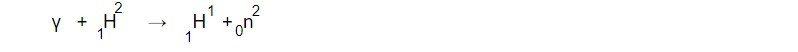5. Radioactive capture: The nuclear reaction in which incident particle is absorbed by nucleus and a compound nucleus is formed in excited state which returns to its ground state by radiating energy in the form of gamma ray photon. For example: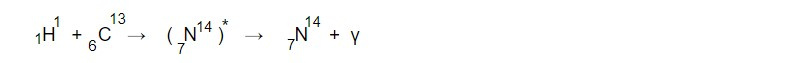6. Direct reaction (stripping and pick up reaction): The nuclear reaction in which one or more particle from the incident particle is captured by the target nucleus and the remainder continues in its way is called as stripping reaction. For example: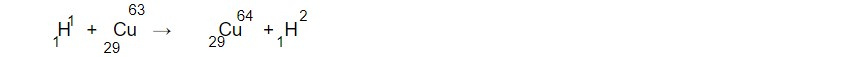The nuclear reaction in which the incident particles picks up one or more particle from the target nucleus is known as pick up reaction. For example: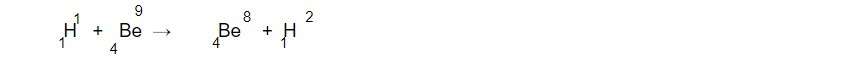7. Fission and spallation reaction: When a projectile particle having small energy strikes on the heavy nuclei, then it breaks up into two nuclei of comparable mass along with the liberation of particles and energy. This reaction is known as fission reaction. For example: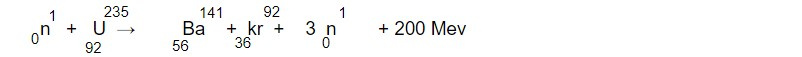If the kinetic energy of incident particle is large, then the target nucleus breaks up into number of smaller nuclei. This reaction is known as spallation reaction.

8. Heavy ion and high energy reaction: If the bombarding particle is heavier than alpha particle then the reaction is called heavy ion reaction.If the energy of bombarding particle is greater than 150 MeV. Then the strange particles, mesons are liberated. This reaction is known as high energy reaction.

9. Fusion reaction: If two smaller nuclei fuse together to form a heavier nuclei along with the release of high energy, then the reaction is known as fusion reaction.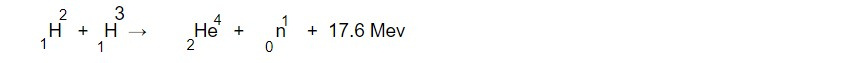Conservation laws in Nuclear reaction

1. Conservation of charge:

The total charge of reactants in a nuclear reaction must be equal to total charge of products. Since the charge can neither be created nor be destroyed.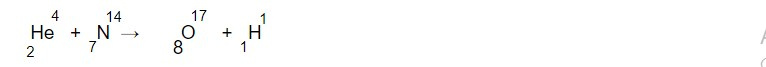2. Conservation of mass number:

Total number of nucleons in the reactant side is equal to total number of nucleons in product side i.e mass number remains conserved.

For example in above reaction

Total mass number of reactants = 4+ 14= 18

Total mass number of products = 17 + 1= 18

3. Conservation of mass and energy:

The sum of rest mass energy and the kinetic energy of reactants must be equal to the total energy (rest mass + K.E) of products. Neither mass nor energy conserve separately in a nuclear reaction.

In the reaction:

a +X -> Y + b + Q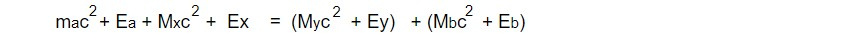where ma, Mx. My, mb -> rest masses

Ea, Ex, Ey, Eb -> K.E of a, X, Y and b particles respectively.

4. Conservation of linear momentum:

The total linear momentum of the reactant particles must be equal to the total linear momentum of product particles.

5. Conservation of angular momentum:

The total angular momentum (J) is given as the sum of spin angular momentum (S) and orbital momentum (L)

i.e J = L + S

Total angular momentum in any nuclear reaction before and after the reaction remains same.

6. Conservation of spin and statistics:

The nuclear spin of reactants remains equal to that of products. So that statistics they follow also remains conserved. if the spin is 1/2, 3/2, 5/2 ....etc then particles obey Fermi Dirac statistics and are called as fermions and if the nuclear spin is 0,1,2,3,....etc. Then the particles obey Bose Einstein statistics and called as Bosons.

7. Conservation of parity:

The parities of reactants is given by the products of particles of individual particles. The parity of reactants is also equal to parity of products.

But parity conservation holds good for strong interaction but this is violated in weak interaction like β decay.

Quantity non conserved in Nuclear reaction

The magnetic dipole moment and electric quadrupole moment do not conserve in nuclear reaction since they depend on distribution of charge, mass and current within the nucleus.

This note is taken from Nuclear Physics, BSC physics, Nepal.

This note is a part of the Physics Repository.